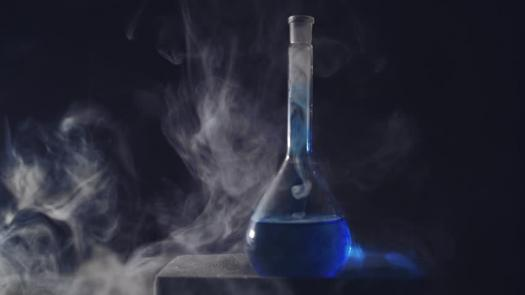# Quiz No.3 '' Heat Content ''

16 Questions | Attempts: 130
ShareSettingsSenior One

• 1.
State whether each of the following are exothermic or endothermic. a-1. H + Cl ---------- HCl                            H=-432 kJ
• A.

Exo

• B.

Endo

• 2.
B-. H2O vapour à H2O liquid
• A.

ENdo

• B.

Exo

• 3.
When two chemicals mix their temperature rises
• A.

Exo

• B.

Endo

• 4.
Plants take in light energy for photosynthesis
• A.

Exo

• B.

Endo

• 5.
What does (delta)H stand for
• A.

The specific heat of substance

• B.

Heat of reaction for chemical reaction

• C.

one calorie given off by a reaction

• 6.
This reaction show the burning of methane in oxygen   Is the reaction exothermic or endothermic overall?
• A.

Exo

• B.

Endo

• 7.
Consider the thermal energy transfer during a chemical process. When heat is transferred to the system, the process is said to be _______ and the sign of H is ________.
• A.

Exo , positive

• B.

Endo , positive

• C.

Exo, negative

• 8.
As element increase its stability , its reaction will be ....................
• A.

Endothermic

• B.

Exothermic

• C.

• 9.
Changing of graphite into diamond is difficult but it undergo type of ................reaction
• A.

Exothermic

• B.

Endothermic

• 10.
Which arrangement is right according to higher heat content ..(H ice , H water , Hvapour)
• A.

Ice ,higher than vapour , higherthan  ice

• B.

Vap higherthan ice lessthan  water

• C.

Ice higherthan water higherthan vapour

• D.

• 11.
Specific heat is sum of all energies in 1mole of any matter
• A.

True

• B.

False

• 12.
Determination of heat content practically is so difficult and has high scientific error
• A.

True

• B.

False

• 13.
Heat content of compound is differ from heat content of element
• A.

True

• B.

False

• 14.
Reaction of baking powder is Exothermic as it take heat from glass after broken the crystal lattice of molecules
• A.

True

• B.

False

• 15.
Heat content of reaction Never Equal Zero
• A.

True

• B.

False

• 16.
Add the bicarbonate soda gradually to avoid overflowing foam and stirring with the thermometer is this reaction is Endothermic Or Exothermic Reaction ?
• A.

Endothermic Reaction

• B.

Exothermic Reaction

• C.Back to top